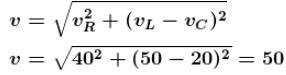Courses

# Alternating Current NAT Level - 1

## 10 Questions MCQ Test Topic wise Tests for IIT JAM Physics | Alternating Current NAT Level - 1

Description
This mock test of Alternating Current NAT Level - 1 for Physics helps you for every Physics entrance exam. This contains 10 Multiple Choice Questions for Physics Alternating Current NAT Level - 1 (mcq) to study with solutions a complete question bank. The solved questions answers in this Alternating Current NAT Level - 1 quiz give you a good mix of easy questions and tough questions. Physics students definitely take this Alternating Current NAT Level - 1 exercise for a better result in the exam. You can find other Alternating Current NAT Level - 1 extra questions, long questions & short questions for Physics on EduRev as well by searching above.
*Answer can only contain numeric values
QUESTION: 1

### In an ac circuit, V and I are given by V = 100 sin 100t volts, I = 100 sin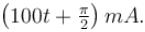The power dissipated (in Watts) in circuit is

Solution: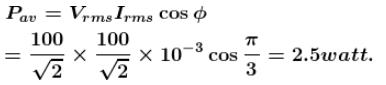*Answer can only contain numeric values
QUESTION: 2

### In the circuit shown below, the ac source has voltage V = 20cos(ωt) Volts with ω = 2000 the amplitude of the current (in Amperes) will be nearest to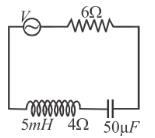Solution: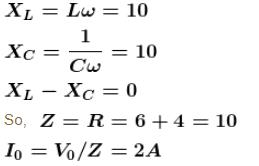*Answer can only contain numeric values
QUESTION: 3

### The power factor of a good choke coil is nearly.

Solution:

Power factor of a good choke coil is 0

*Answer can only contain numeric values
QUESTION: 4

A coil of  200Ω resistance and 1.0H  inductance to an ac source of frequency 200/ 2π Hz. Phase angle (in degree) between potential and current will be

Solution: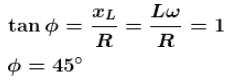*Answer can only contain numeric values
QUESTION: 5

When 100V  D.C. is applied across solenoid a current of 1.0A flows in it. When 100 V A.C. is applied across the same coil the current drops to 0.5A. If the frequency of the A.C. source is 50Hz, the impedance (in Ω) of the solenoid is.

Solution: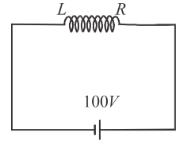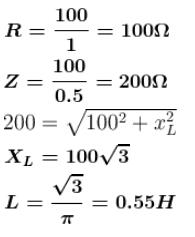*Answer can only contain numeric values
QUESTION: 6

In the figure shown, a current of 2A flows in the circuit. The impedance (in Ω) of the circuit is

Solution: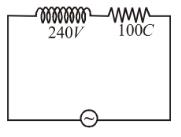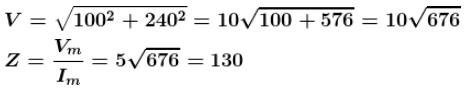*Answer can only contain numeric values
QUESTION: 7

In an ac circuit the current is given by i = 0.5sin(314t + 60º)mA. The peak to peak value of current is (in mA).

Solution:

Peak to Peak value of current is 1mA

*Answer can only contain numeric values
QUESTION: 8

A steady dc current of 2.83A is flowing in a resistance wire. The rms value of the current (in Amperes) is :

Solution:

The rms value of the current is 2.83A

*Answer can only contain numeric values
QUESTION: 9

In series LCR circuit the voltage across R, L and C are shown in figure. The Voltage (in Volts) of applied source is :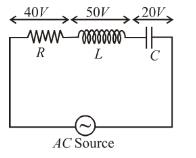Solution: